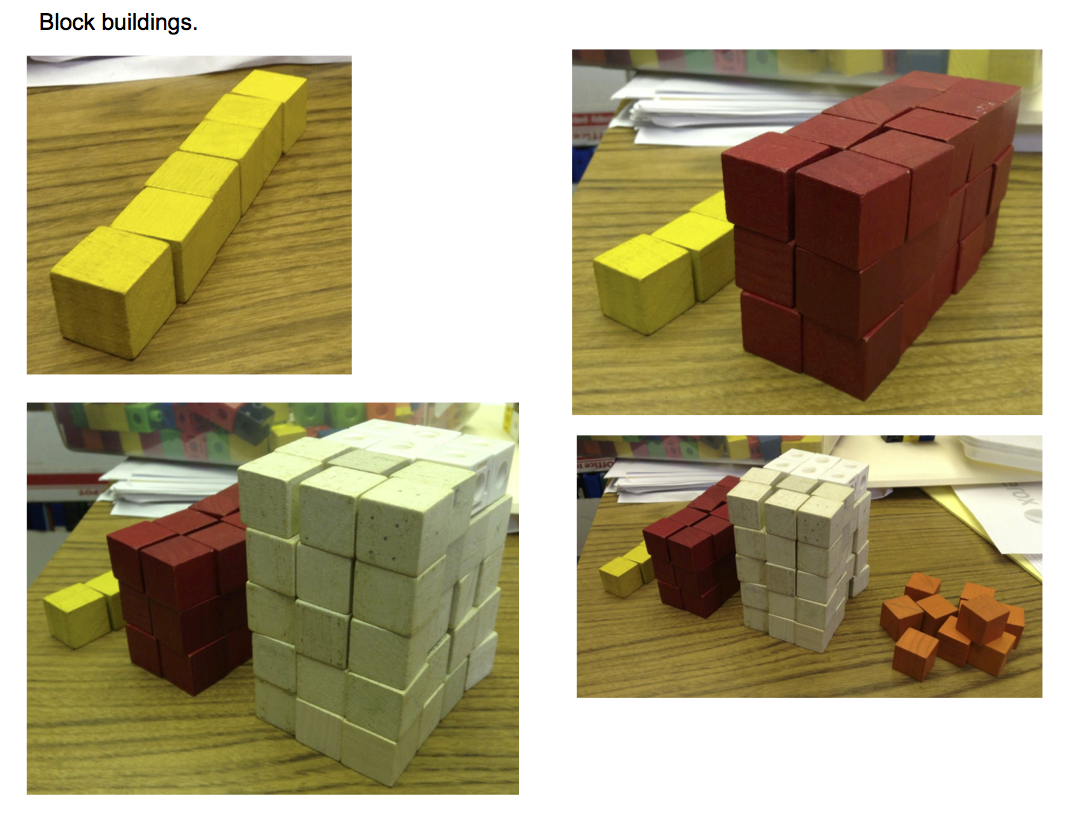1. How many blocks to make these buildings?

1. How many blocks to make the next building? How do you know?
Draw and label a picture with lengths.

1. What is the pattern – or what are some of the patterns? How would you describe it?

1. Will the pattern continue indefinitely? Is there going to be a biggest building? What do we mean by biggest – tallest or most cubes?

1. Can we describe it in algebraic terms?

1. Make a table of the data. Examine the differences. Extend the table.

1. Volume of rectangular boxes uses the height width and length, so… Find an algebraic rule for the height. Another for the width. Another for the height. Combine for a volume rule.

1. Graph the volume rule. What can you tell? How does it make sense?

1. Next level stuff: can you see the rule in the pictures?

1. How is algebra like generalizing?